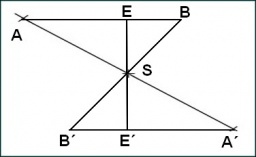# ​​Calculate 6706

Given a triangle KLM points K [-3.2] L [7, -3] M [8.5]. ​​Calculate the side lengths and perimeter.

k =  8.0623
l =  11.4018
m =  11.1803
o =  30.6444

### Step-by-step explanation:

Try calculation via our triangle calculator.Did you find an error or inaccuracy? Feel free to write us. Thank you!

Tips for related online calculators